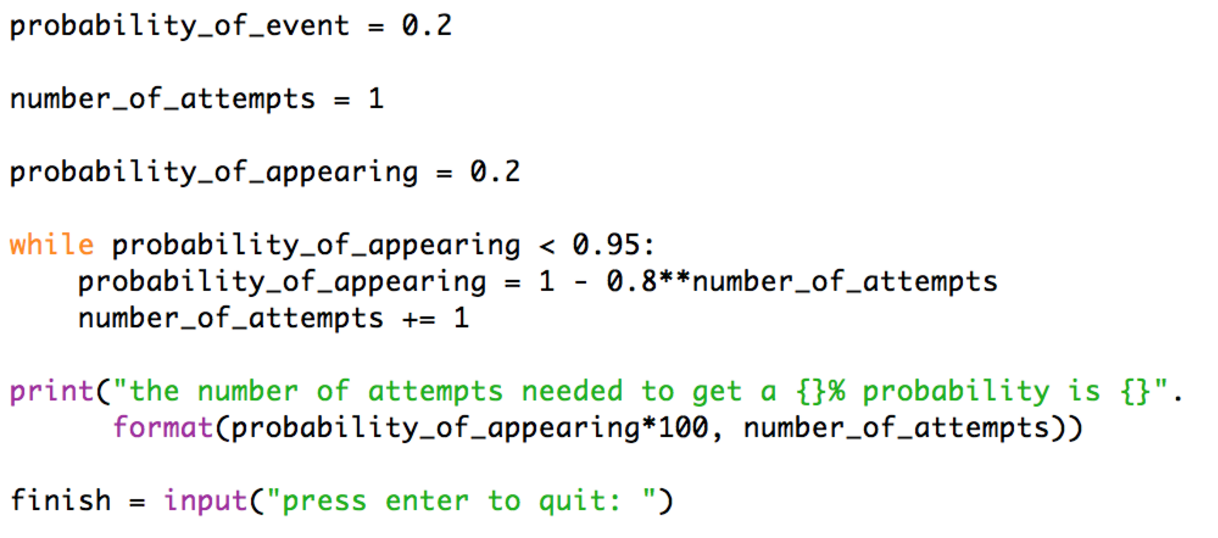# How to construct a mathematical model

coAs it is for many doctors and nurses maths and mathematical modelling isn’t covered much in education. This doesn’t mean that the undergraduate education needs an overhaul. There is a lot of learn when training to be a nurse or doctor. However, when doctors and nurses start finding their way in the clinical setting some have an interest in data, data analysis, and modelling. These people need extra support. I only realised how bad my math skills were when I went part time in the Emergency department and completed a physics degree. It was only then that I understood the difference between mathematical modelling and statistics. If the difference is unclear to you please read: Why machine learning in medicine. I was then told that there are too many variables to account for most outcomes in medicine. I believed this until I learnt how to code and discovered machine learning. Again read the machine learning post if you are not familiar on how machine learning is applied to medicine. As a doctor or nurse developing a mathematical model can seem very daunting as there are no clear rules unlike the approach to clinical statistics. However, if you follow this process step by step you will not get lost and are more likely to produce something of use.

Step 1.

Define the purpose. This may seem a little basic but it’s important. We need to define a measurable outcome that’s useful. For this example, we will say that our stats how 20% of patients with x disease have event y happen to them. We want to produce a model that predicts how many patients we have to see with disease x until we have a 95% probability that at least one of them will have event y.

Step 2.

Make assumptions. This may sound a little sketchy but it is the only way you can reason anything. You have to make assumptions. The crazier the assumptions the less accurate your model will be. The laws of physics are assumptions when developing models of physical systems. In our case, we could assume that adding probabilities would be a rational assumption. If there’s a 20% chance that I’ll see event y with one patient with disease x there is a 40% chance of seeing event y when seeing 2 patients with disease x, doubling the patient number doubles the chance. This seems rational at face value but it is a terrible model. If you see 5 patients with disease x you will have 100% chance of event y happening. Seeing 6 patients would result in a 120% chance. Lets make the assumption that the probability of y not happening plus the probability of y happening is 100%. Lets also make the assumption that the same event happening twice in a row is computed by squaring it. So coming across one patient the probability of y happening is 0.2, coming across the second patient and y also happening to them is 0.2 squared. This is established probability theory.

Step 3.

Do the math. Now we have two assumptions lets build our model. From the first assumption we have:

Y_not_happening + Y_happening = 1

This gives:

Y_happening = 1 – Y_not_happening

Now considering our second assumption we every time we see a new patient we have to compute the probability of it not happening again and again. When multiplying something by itself again and again you can save time by raising it to the power of. We also know that 0.2 + 0.8 = 1 thus the probability of y not happening is 0.8 so our model is:

Y_happening =  1 – (0.8)**n

n is the number of patients you have seen. If you keep raising a number lower than 1 to a higher power it will get smaller so the more patients with disease x we see the higher the probability that event y will happen.

Step 4

Write the code!!!!! This is the exciting part. Don’t worry if you haven’t written code before. I’ve written the program in python 3, simply download python 3.5 for free [link] and copy this code out. If you have followed me through the modeling steps you should be able to grasp what’s being coded. Python is a high-level language and easy/quick to develop in.Once you have copied the code into a new python file and saved it run it. It’s a very basic program. It’s designed this way so you don’t feel intimidated and motivated to develop your coding skills further. With a little more coding study you can do a range of calculations in seconds and get it to plot the probability for each patient interaction as the increase in probability will not be linear (straight).

The result is that you have to see 15 patients with x disease in order to get a 95% probability that you will come across at least one y event. Very different to 5 patients resulting in 100%. Probability is very counter-intuitive. If you feel a little worried after this exposure do not worry. Doctors and nurses are famous for having a poor grasp on probability concepts. Gigerenzer’s research showed that 2 thirds of medical consultants failed basic probability questions. Keep modeling and keep coding and you will get better.## maxwellflitton View All →

I help clinicians get to grips with coding and tech, I also code for a financial tech firm

This site uses Akismet to reduce spam. Learn how your comment data is processed.